Square Roots 2
Set 2 (10 Questions)
Students use exponents, powers, and roots, and use exponents in working with fractions
From Mr. Anker Tests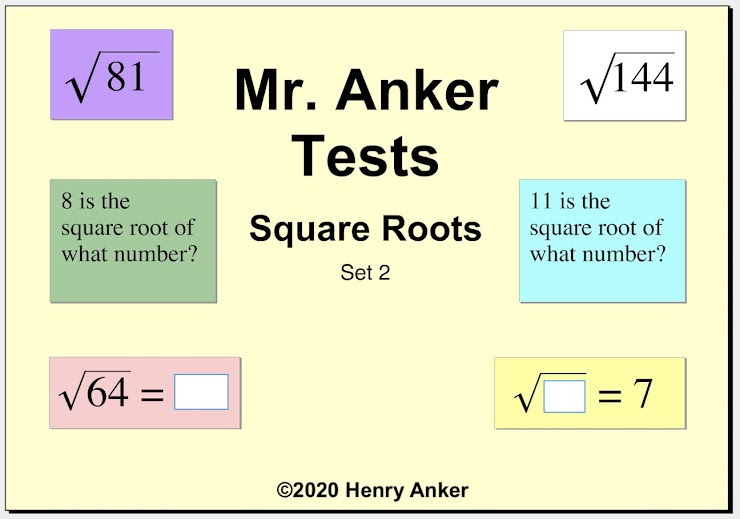Square Roots Help Slide 1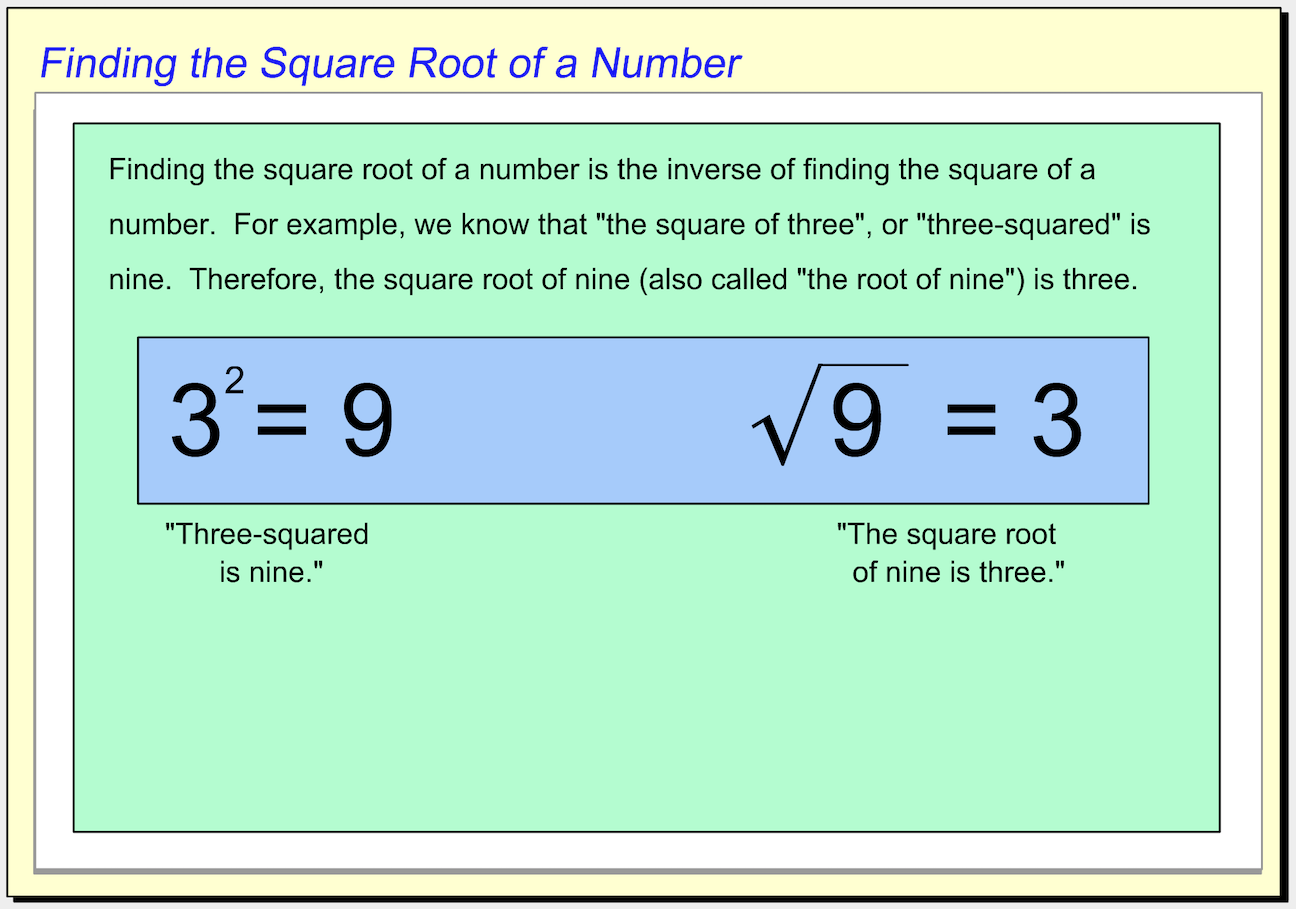Square Roots Help Slide 2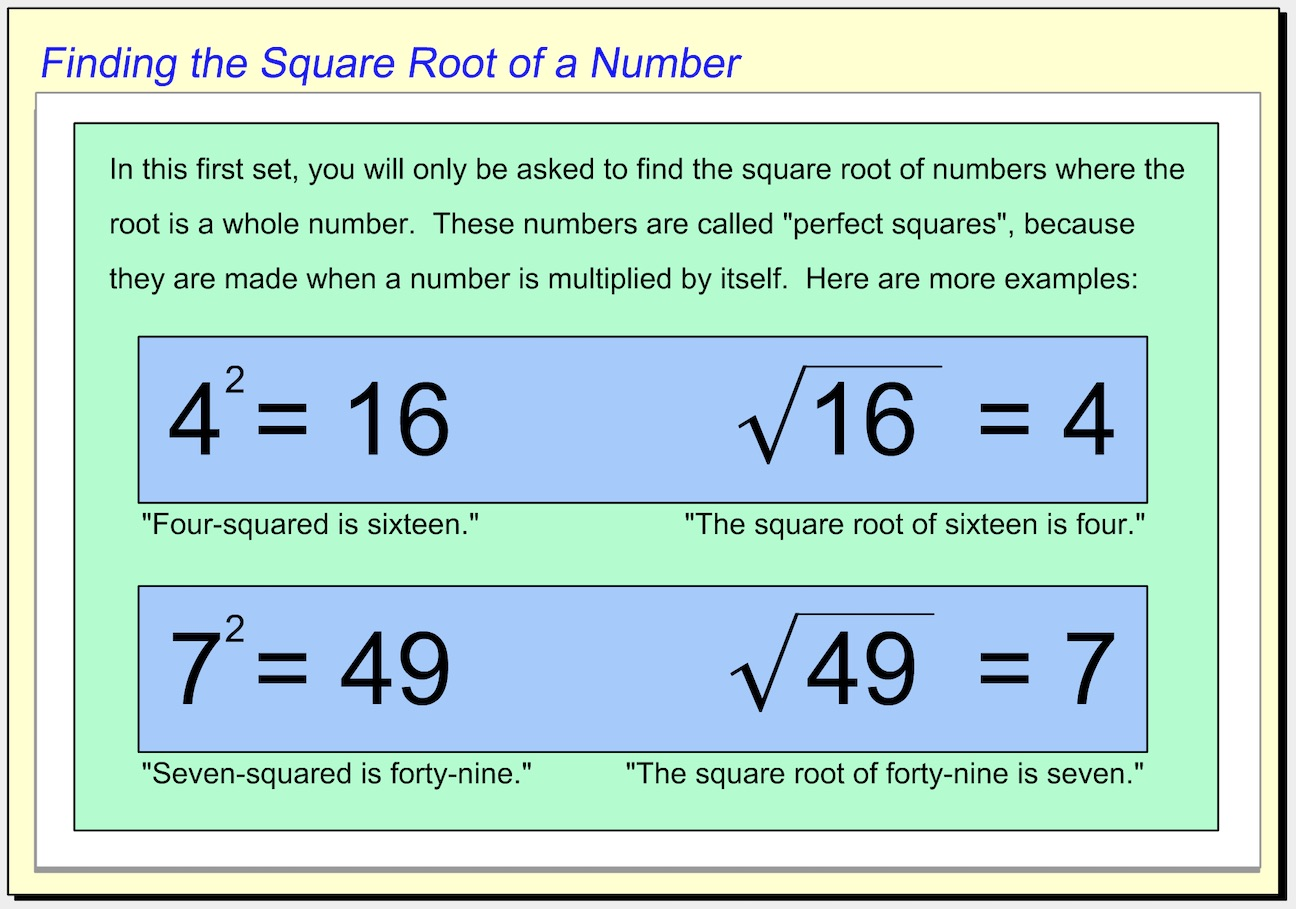Square Roots Help Slide 3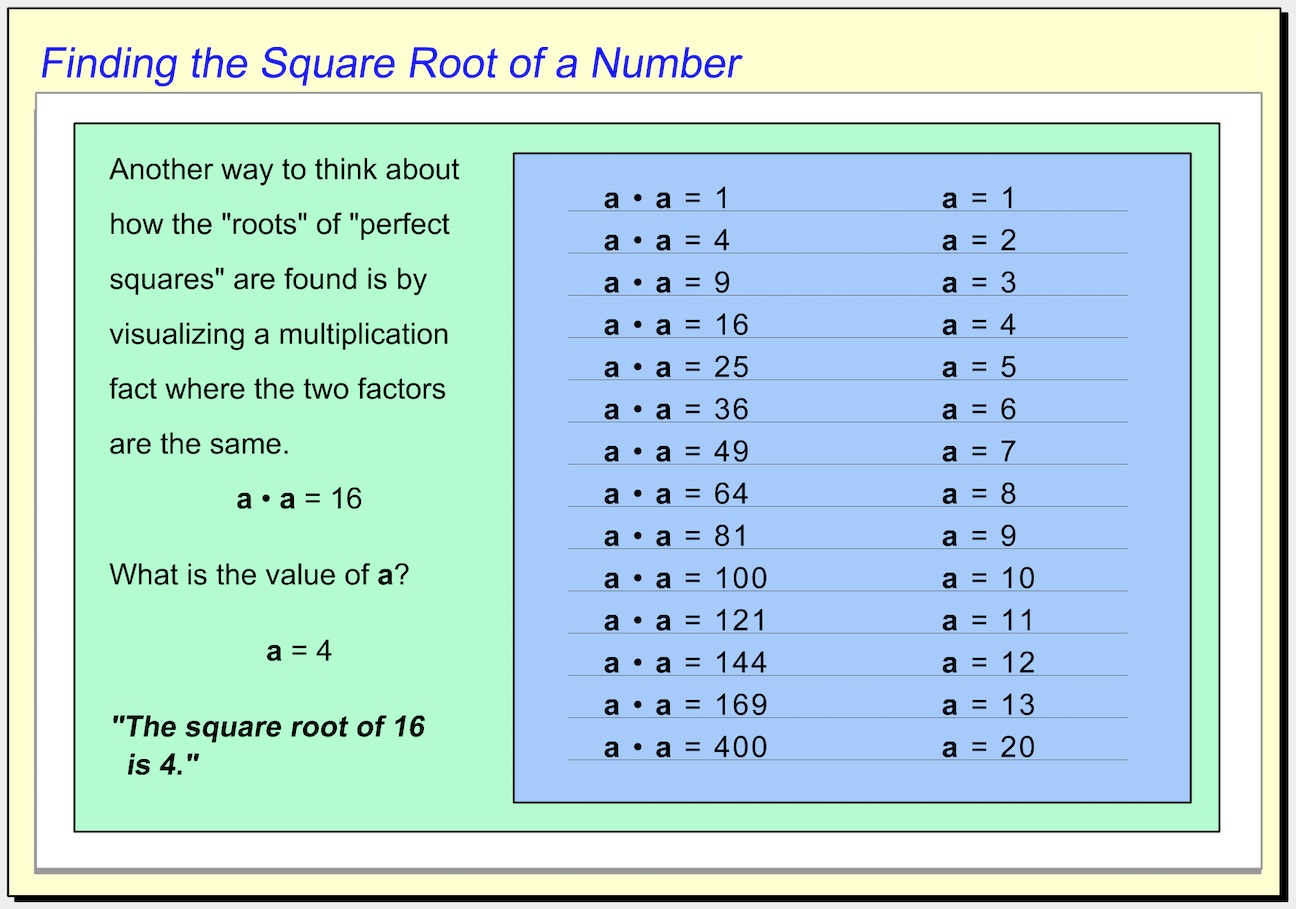Square Roots Help Slide 41.  Which choice shows the value of the square root displayed? *
1 point2.  Which choice shows the value of the square root displayed? *
1 point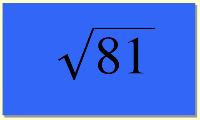3.  Which choice shows the value of the square root displayed? *
1 point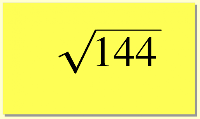4. Use the dropdown menu below to make this statement true: "Forty-four divided by eleven is __________ the square root of 16." *
1 point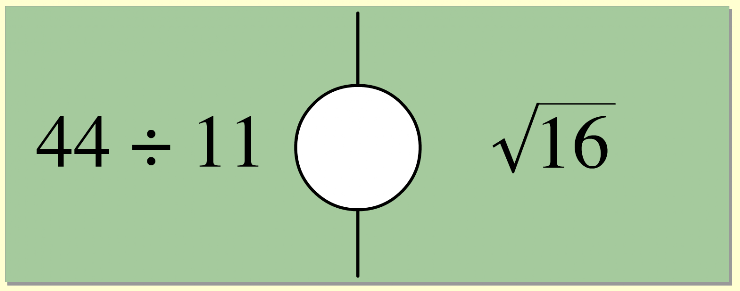5. Which number goes in the box to make the equation true? *
1 point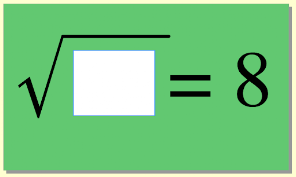6.  Use the dropdown menu below to make this statement true: "The square root of 36 is __________ 5." *
1 point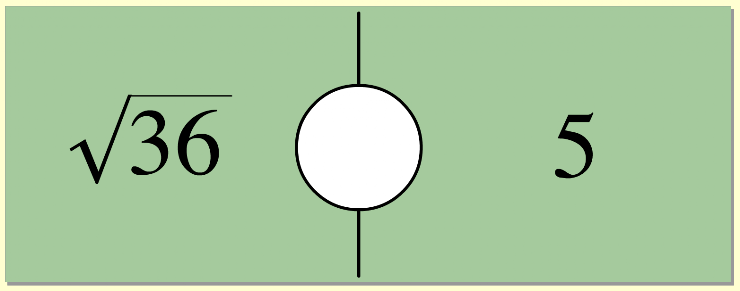7.  Which choice shows the value of the square root displayed? *
1 point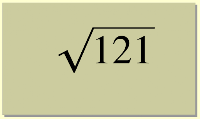8.  Use the dropdown menu below to make this statement true: "Five plus one is __________ seven minus the square root of one." *
1 point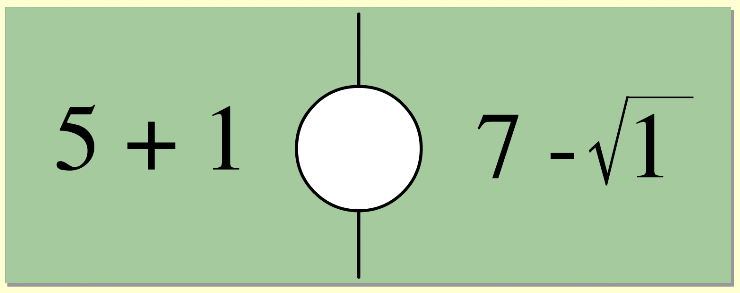9.  On the line below, enter the equal value as a whole number. *
1 point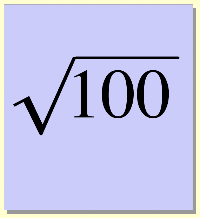10.  Use the dropdown menu below to make this statement true: "The product of 2 times 12 is __________ the square root of 144." *
1 point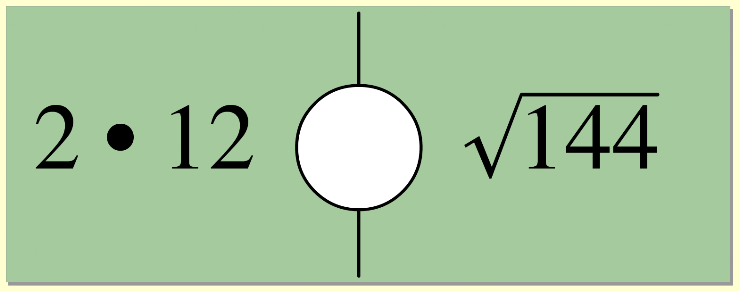Submit
Clear form